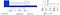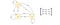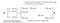# Machine Learning & Linear Algebra — Eigenvalue and eigenvectorPhoto by Yuiizaa September

Eigenvalue and eigenvector are probably one of the most important concepts in linear algebra. Who can expect a simple equation like Av = λv is so significant? From machine learning to quantum computing, many problems can be solved by finding the eigenvalue and eigenvectors of a matrix. In this article, we will discover why it is so important and how we can apply it. We will also take a look into the Google PageRank, a core part of the Google search engine, to see how it is related to eigenvectors.

By definition, scalar λ and vector v are the eigenvalue and eigenvector of A if

Visually, Av lies along the same line as the eigenvector v.

Ax does not usually equal to λx. Only some exceptional vectors satisfy the condition. Here are some eigenvector examples.

If the eigenvalue is greater than one, the corresponding Avᵢ will expand. If it is smaller than one, it will shrink.

# Application

But before getting into details, let’s pause and appreciate the beauty of such an abstract concept first. In specific, many problems can be modeled with linear algebra with solutions derived from eigenvalues and eigenvectors.

Let’s start with an abstract example first before getting into a real billion-dollar idea — Google’s PageRank. In many systems, we can express its states in a vector with their rates of change linearly depend on the current states (e.g. the population growth rate is linearly depending on the current population and GDP.). The general equation is

where u composes of n properties. So let’s take a guess on the solution for u(t) that satisfies the equation above. Since the derivative of an exponential function equals itself, we can start the guess with an exponential function of t and multiply it with a vector x. The output will also be a vector and we will compute the value of x and λ next.

From the calculation above, our guess will satisfy du/dt = Au if x and λ are the eigenvectors and eigenvalues of A. i.e.

In my systems, the problem can be rephrased and solved by eigenvalues and eigenvectors.

Next, let’s find its complete solution. Our first order derivative equation is a linear function.

For linear functions, the complete solution is the linear combination of particular solutions. If u and v are the solutions, C₁u + C₂v is also the solution. From our previous example with eigenvalues λ = 4, -2 and -2, the complete solution will be

If a system will reach a stable state, then all eigenvalues have to be negative. Otherwise, the non-negative term will change continuously. At time t=0, we can measure the initial state u(0), say [u₀₁, u₀₂, u₀₃]ᵀ, and solve the constant C₁, C₂, and C₃.

Let’s illustrate the idea with a harmonic oscillator. We pick this example because the harmonic oscillator and its close cousin, the quantum harmonic oscillator, are fundamental in studying particle physics, quantum mechanics, string theory or physics in general. Let’s start with the famous F=ma equation and use eigenvalues and eigenvectors to solve the second-order derivative. Since we do have the freedom to pick the unit for mass, physicists often set m=1 to simplify the discussion, i.e.

We reformulate the harmonic oscillator problem into the matrix form in the bottom right below.damping harmonic oscillator

This has the same form as our last example and therefore, we can use the eigenvalues and eigenvectors of A to form the complete solution.

This is not an isolated example in demonstrating the power of eigenvalues. Nature seems to have an eigenvector cookbook when making its design. The famous time-independent Schrödinger equation is expressed with eigenvalues and eigenvectors. All observed properties are modeled by eigenvalues in quantum mechanics. They are many other examples including machine learning. One of the biggest eigenvector computed is Google PageRank which is the core part of the Google search.

Fundamentally, many systems can be modeled as

Let’s study the time sequence model below for the purpose of machine learning.

First, we assume the initial state u₀ to be an eigenvector of A. Therefore, its future states can be computed as

This equation can be simplified by replacing the power of a matrix (Aᵏ) with the power of a scalar that is much easy to compute. Next, let’s generalize the problem to include any initial state. Consider A has n linearly independent eigenvectors that form a basis of Rⁿ. We can decompose any vector of Rⁿ into this basis. Again, we can simplify the time sequence calculation by computing the power of the eigenvalue again.

If a system will reach a stable state, we should again expect λᵢ to be smaller or equal to 1. In addition, to compute this stable state, we can ignore terms with λᵢᵏ smaller than 1. As time goes by, these terms diminish to 0. Hence, to find a stable state, we can just focus on eigenvectors associated with λᵢ = 1.

Let’s discuss a real multi-billion idea to realize its full potential. Let’s simplify our discussion and assumes the whole internet contains only three web pages. The element Aof a matrix A is the probability of a user going to page i when the user is on page j.

Given a specific page, if we sum over all the possibilities of the next page, it equals 1. i.e., all columns of A sum up to 1.0 and this kind of matrix is called the stochastic matrix, transition matrix or Markov matrix.

Markov matrix has some important properties. The result of Ax or Aᵏx always sums up to one with its columns. The right-hand term is the chance of being on pages 1, 2 and 3 respectively after each click. So it is obvious that it should sum up to one.

Any Markov matrix A has an eigenvalue of 1 and the absolute value of other non-one eigenvalues to be smaller than one. This behavior is very important. In our example,

For a Markov matrix, we can choose the eigenvector for λ=1 to have elements sum up to 1.0. So vectors v0 can be decomposed using the eigenvectors of A with c₁ equals to 1 below.

Since u₁, u₂, …, and un are eigenvectors, Aᵏ can be replaced by λᵏ. Except for eigenvalue λ=1, the power of the eigenvalue (λᵏ) for a Markov matrix will diminish. So the system reaches a steady-state that approaches the eigenvector u₁ regardless of the initial state v0. The steady-state will equal the eigenvector u₁ as shown below.

From our example, the chance we land on pages 1, 2 and 3 are about 0.41, 0.34 and 0.44 respectively. This concept has many potential applications. For instance, many problems can be easily modeled with Markov processes and a Markov/transition matrix.Markov process and the transition matrix

# Google PageRank

I am not exaggerating when I say this is a billion-dollar idea. The PageRank algorithm named after Google co-founder Larry Page is based on a similar concept. It is the first Google search ranking algorithm even it is heavily modified now with added ranking algorithms to improve user experience and to avoid manipulation.

The core idea can be conceptualized as the following. PageRank outputs a probability distribution of pages that you may land on by following links on a web page after many many clicks. This probability will act as the ranking of a Web page. When many pages link to your Web page, Google will rank it higher considering it as a good indicator of popularity.

In our previous example, we have not discussed ways to derive the values in the Markov matrix. For page rank, we compute the values as the sum of other page ranks linked to this page divided by its total number of outbound pages.

Mathematically, PageRank tries to solve the PageRank vector R (an eigenvector) in the following equation. It initializes R with equal probability at the beginning and performs the calculation iteratively until it reaches a steady-state.Modified from Wikipedia

The matrix element lᵢⱼ above is the ratio between the number of the outbound page from page j to page i to the total number of the outbound page of j. Each column of l adds up to 1 and it is a Markov matrix. This equation has an enormous similarity with the example we discussed before if we ignore the damping factor d. This factor is introduced because we will not have a random walk to take forever steps.

For Google, they do not compute the eigenvectors directly. In our previous example, the power of A converges very fast which the column of converge to eigenvector u₁ already.

The PageRank paper has demonstrated that with 322 million page links, the solution converges to a tolerable limit in 52 iterations. So the solution scales unexpectedly well.

The Markov matrix leads us to the equation below which the steady-state depends on one principal component.

In machine learning, information is tangled in raw data. Intelligence is based on the ability to extract the principal components of information inside a stack of hay. Mathematically, eigenvalues and eigenvectors provide a way to identify them. Eigenvectors identify the components and eigenvalues quantify its significance. As a preview, the equation below decomposes information in A into components. We can prioritize them based on the square root of eigenvalues and ignore terms with small α values. This reduces the noise and helps us to extract the core information in A. (Details on a later article.)

# Solution

I hope you can see the beauty of Ax = λx for now. Let’s see how can we solve it. Eigenvalues and eigenvectors can be calculated by solving (A - λI) v = 0. To have a solution other than v=0 for Ax = λx, the matrix (A - λI) cannot be invertible. i.e. it is singular. Its determinant is zero. det(A - λI) = 0 is called the characteristic polynomial and the eigenvalues are the root of this polynomial.

Example

The eigenvalues are:

Apply Av = λv, we solve:

Let’s detail the step with a more complicated example,

To find the eigenvalue λ,

The possible factors for 16 are 1, 2, 4, 8, 16.

Let’s calculate the eigenvector for eigenvalue λ = 4 through row reduction.

We have three variables with 2 equations. We set x₃ arbitrary to 1 and compute the other two variables. So for λ=4, the eigenvector is:

We repeat the calculation for λ=-2 and get

With 3 variables and 1 equation, we have 2 degrees of freedom in our solution. Let’s set the value to one in one of the degrees of freedom while other(s) to 0. i.e. setting x₂=1, x₃=0, and x₂=0, x₃=1 separately, the calculated eigenvectors will be:

Note that the solution set for eigenvalues and eigenvectors are not unique. we can rescale the eigenvectors. We can also set different values for x₂, x₃ above. Hene, it is possible and desirable to choose our eigenvectors to meet certain conditions. For example, for a symmetric matrix, it is always possible to choose the eigenvectors to have unit length and orthogonal to each other.

In our example, we have a repeated eigenvalue “-2”. It generates two different eigenvectors. However, this is not always the case — there are cases where repeated eigenvalues do not have more than one eigenvector.

# Diagonalizable

Let’s assume a matrix A has two eigenvalues and eigenvectors.

We can concatenate them together and rewrite the equations in the matrix form.

We can generalize it into any number of eigenvectors as

since

where V concatenates all the eigenvectors and Λ (the capital letter for λ) is the diagonal matrix containing the eigenvalues.

A square matrix A is diagonalizable if we can convert it into a diagonal matrix, like

i.e.,

An n × n square matrix is diagonalizable if it has n linearly independent eigenvectors. If a matrix is symmetric, it is diagonalizable. If a matrix does not have repeated eigenvalue, it always generates enough linearly independent eigenvectors to diagonalize a vector. If it has repeated eigenvalues, there is no guarantee we have enough eigenvectors. Some will not be diagonalizable.

# Eigendecomposition

If A is a square matrix with N linearly independent eigenvectors (v₁, v₂, … & vn and corresponding eigenvalues λ₁, λ₂, … & λn), we can rearrange

into

For example,

However, there are some serious limitations on eigendecomposition. First, A needs to be a square matrix. Second, V may not be invertible. As a preview, SVD solves both problems and SVD decomposes a matrix into orthogonal matrices which are easier to manipulate. But for matrices like symmetric matrices, this is not a problem. The power of a diagonalizable matrix A is easy to compute.

This demonstrates the power of decomposing a matrix that can be manipulated easily.

To recap, for states that can be expressed as

Aᵏ can be computed with eigenvalues.

# Properties of eigenvalue & eigenvectors

• Ax lies on the same line as the eigenvector x (same or opposite direction).
• The sum of eigenvalues equals the trace of a matrix (sum of diagonal elements).
• The product of eigenvalues equals the determinant.
• Both conditions above serve as a good insanity check on the calculations of eigenvalues.
• If no eigenvalue is repeated, all eigenvectors are linearly independent. Such an n × n matrix will have n eigenvalues and n linearly independent eigenvectors.
• If eigenvalues are repeated, we may or may not have all n linearly independent eigenvectors to diagonalize a square matrix.
• The number of positive eigenvalues equals the number of positive pivots.
• For Ax = λx,
• If A is singular, it has an eigenvalue of 0. An invertible matrix has all eigenvalues non-zero.
• Eigenvalues and eigenvectors can be complex numbers.
• Projection matrices always have eigenvalues of 1 and 0 only. Reflection matrices have eigenvalues of 1 and -1.

# More thoughts

Eigenvalues quantify the importance of information along the line of eigenvectors. Equipped with this information, we know what part of the information can be ignored and how to compress information (SVD, Dimension reduction & PCA). It also helps us to extract features in developing machine learning models. Sometimes, it makes the model easier to train because of the reduction of tangled information. It also serves the purpose to visualize tangled raw data. Other applications include the recommendation systems or financial risk analysis. For example, we suggest movies based on your personal viewing behavior and others. We can also use eigenvectors to understand the correlations among data. Develop trends of the information and cluster information to find the common factors, like the combination of genes that triggers certain kind of disease. And all of them start from the simple equation:

Matrices are not created equally. It is important to know the properties of some common matrices in machine learning. Next, we will take a look at it.

Deep Learning